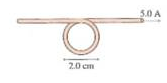# Problem: What are the strength and direction of the magnetic field at the center of the loop?

###### FREE Expert Solution

Magnetic field due to a loop:

$\overline{){\mathbf{B}}{\mathbf{=}}\frac{{\mathbf{\mu }}_{\mathbf{0}}\mathbf{I}}{\mathbf{2}\mathbf{r}}}$

Magnetic field due to a straight conductor:

$\overline{){\mathbf{B}}{\mathbf{=}}\frac{{\mathbf{\mu }}_{\mathbf{0}}\mathbf{I}}{\mathbf{2}\mathbit{\pi }\mathbf{r}}}$

The magnetic field at the center of the loop is the sum of the field due to the loop and a straight conductor.

93% (92 ratings)###### Problem Details

What are the strength and direction of the magnetic field at the center of the loop?Frequently Asked Questions

What scientific concept do you need to know in order to solve this problem?

Our tutors have indicated that to solve this problem you will need to apply the Magnetic Field Produced by Loops and Solenoids concept. You can view video lessons to learn Magnetic Field Produced by Loops and Solenoids. Or if you need more Magnetic Field Produced by Loops and Solenoids practice, you can also practice Magnetic Field Produced by Loops and Solenoids practice problems.

What professor is this problem relevant for?

Based on our data, we think this problem is relevant for Professor Jerousek's class at UCF.